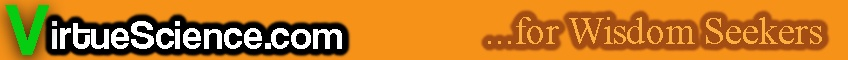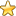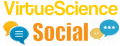Database of Number Correlations The purpose of this section is to discover unexpected connections between various material and occult phenomena. A great resource for mathematicians. Notable Events for over 2000 years of history: Discover unusual synchronicities in World History. 2500 plus pages.
Great Mathematician Ramanujan was helped by?
Which Number is both a Square Number and a Square Pyramidal Number?
I am an odd number. Take away one letter and I become even. What number am I?
Infinity plus infinity equals....?
Has the Use of Calculators Weakened Human Minds?
How Many Mathematical Axioms are There?
What is the Impact of Artificial Intelligence on Mathematics?
What is the Most Interesting Unsolved Maths Question?
Do you have a Favourite Number?
The Database of Number Correlations
The Main Number Sections:
0 - 500 | 501 - 1000 | 1001 - 1500 | 1501 - 2000 | 2001 - 2500
Number Articles | Higher Numbers | Random Number
You are here: HomeThe Database of Number Correlations# Partition Theory

Partition theory is a fundamental area of number theory. It is concerned with the number of ways that a whole number can be partitioned into whole number parts.

5 for example can be partitioned in 7 ways thus:
11111, 2111, 221, 311, 32, 41, 5.
The permutations of these 7 partitions add up to 16 thus:
 11111 =1 permutation 2111 =4 permutations 221 =3 permutations 311 =3 permutations 32 =2 permutations 41 =2 permutations 5 =1 permutation

A partition is a way of writing an integer n as a sum of positive integers where the order of the parts is not significant.
 Number Partitions Order counts 0 1 1 1 1 1 2 2 2 3 3 4 4 5 8 5 7 16 6 11 32 7 15 64 8 22 128 9 30 256 10 42 512 11 56 1024 12 77 2048 13 101 4096 14 135 8192 15 176 16384 16 231 - 17 297 - 18 385 - 19 490 - 20 627 - 21 792 - 22 1002 -
http://home.att.net/~numericana/data/partition.htm Partition Numbers for 0 to 4096 (site down?)

# 5, 7, 11 Congruences: Properties and Meanings

Starting with 4, the number of partitions for every 5th integer is a multiple of 5-eg the number of partitions for 9 is 30 and for 14 is 135.

Starting with 5, every 7th integer is a multiple of 7.

Starting with 6, every 11th integer is a multiple of 11.

# Other Relationships: Properties and Meanings

Number of partitions of n into an even number of distinct parts equals the number of partitions of n into an odd number of distinct parts-except when n is a Pentagonal Number.(Franklin?)

Number of partitions of n into parts not divisible by 3>than the number of partitions of n into parts none of which occurs more than twice.

The number of odd partitions of n=the number of distinct partitions of n.

The total number of 1s that occur among all unordered partitions of a positive integer is equal to the sum of the numbers of distinct members of those partitions.

SEQUENCE: 0, 1, 3, 6, 12, 20, 35, 54, 86, 128, 192, 275, 399, 556, 780, 1068, 1463, 1965, 2644, 3498, 4630, 6052, 7899, 10206, 13174, 16851, 21522, 27294, 34545, 43453, 54563, 68135, 84927, 105366, 130462, 160876, 198014, 242812, 297201, 362587, 441546, 536104, 649791, 785437, 947812, 1140945, 1371173, 1644136, 1968379, 2351597, 2805218, 3339869, 3970648, 4712040, 5584141, 6606438, 7805507, 9207637 ...
The total number of parts in all partitions of n. Also sum of all large parts of all partitions of n.

SEQUENCE: 0, 0, 1, 3, 7, 13, 24, 39, 64, 98, 150, 219, 322, 455, 645, 892, 1232, 1668, 2259, 3008, 4003, 5260, 6897, 8951, 11599, 14893, 19086, 24284, 30827, 38888, 48959, 61293, 76578, 95223, 118152, 145993, 180037, 221175, 271186, 331402, 404208, 491521...
The number of "+" signs needed to write the partitions of n.

Number of partitions of n into distinct parts >= 2....number of partitions of n into odd parts.
1, 1, 1, 2, 2, 3, 4, 5, 6, 8, 10, 12, 15, 18, 22, 27, 32, 38, 46, 54, 64, 76, 89, 104, 122, 142, 165, 192, 222, 256, 296, 340, 390, 448, 512, 585, 668, 760, 864, 982, 1113, 1260, 1426, 1610, 1816, 2048, 2304, 2590, 2910, 3264, 3658, 4097, 4582, 5120, 5718
http://www.research.att.com/~njas/sequences/?q=distinct+parts&sort=0&fmt=0&language=english&go=Search

You are here: HomeThe Database of Number CorrelationsThe Main Number Sections:
0 - 500 | 501 - 1000 | 1001 - 1500 | 1501 - 2000 | 2001 - 2500
Number Articles | Higher Numbers | Random NumberCheck out the latest Number / Mathematics Forum Topics:Hi, I am James Barton an independent truth seeker.
VirtueScience is a unique philosophy and set of tools which I have developed over many years. It combines deep new insights with ancient wisdom.
Main Article Sections